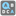• # Common words

## Question related to missionCommon Words

```def checkio(first, second):
a = set(first.split(","))
b = set(second.split(","))
return ",".join(sorted(a.intersection(b))

#These "asserts" using only for self-checking and not necessary for auto-testing
if __name__ == '__main__':
assert checkio("hello,world", "hello,earth") == "hello", "Hello"
assert checkio("one,two,three", "four,five,six") == "", "Too different"
assert checkio("one,two,three", "four,five,one,two,six,three") == "one,three,two", "1 2 3"
```

I get an error after checking, in PyCharm i get a syntax error like

```if __name__ == '__main__':                             ^
SyntaxError: invalid syntax"
```